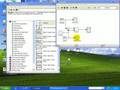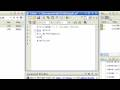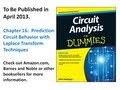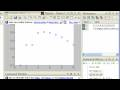UniversitiesLOGINCONTACTRelated Videos
ViewMATLAB Tutorial: Getting Started with MATLABMatlab Tutorial - Amplitude ModulationMATLAB Function: Writing a MATLAB ProgramMATLAB Tutorial Part 2: Basic OperatorsMatlab Tutorial - Partial Fraction Expansion and Inverse Laplace Transform ExampleSimulink / Matlab Tutorial and Example - Low Pass Filter (Part 1)Matlab Tutorial - Partial Fraction Expansion and Inverse Laplace Transform Example (Shorter Version)MATLAB Tutorial Part 3: Functions and %27plot%27MATLAB Tutorial Part 6: Example (Plastic Bottle on Water)MATLAB Editor: Developing MATLAB Code Rapidly with CellsMATLAB Tutorial Part 8: Bisection Method (Root finding)MATLAB Tutorial Part 5: MatricesIP&PR IMAGE PROCESSING AND PATTERN RECOGNITION Videos 12 eBooks 15 Magazines 4 Companies 1
 Rate This Video
 Video Rating:Coments